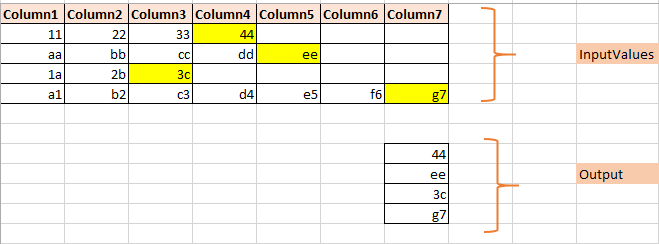# Get the data from last column from an excel sheet when the column number is not known

Hi,
I am trying to get the data from the last column of every row from an excel sheet… but since the column number is not known and every row has different different number of columns, I am unable to do this.
Will appreciate any help.

Hey, use a for each row activity and try this in the write line activity.

datatable(datatable.Rows.Count-1)(datatable.Columns.Count-1).ToString

Replace “datatable” field with your datatable name.
Hope it helps!

@rachrahul2 hey, thanks for your solution but it is showing an exception:
“Read Range: Object reference is not set to an instance of an object”.

Maybe datatable is not suitable for the number of columns is dynamic.
My thought is: execute a macro to get every last column to output into the excel

Here is the sample:

Sub doCalucLastColumn()

Range(“K2”).Select
For i = 1 To 7
Cells(i + 1, 11) = getLastColumn(i + 1)
Next i

End Sub

Function getLastColumn(intRowNum As Integer) As Integer

getLastColumn = ActiveSheet.Cells(intRowNum, Columns.Count).End(xlToLeft).Column

End Function

The macro name is “doCalucLastColumn” .

Neha, Correct me if Iam wrong with my understanding

RequirementSolution: (Using Linq)

### 1) From Query…

``````List lastColumnValues = (From row In dt.Select()
Select row.ItemArray.Where(Function(x) Not String.IsNullOrEmpty(x.Tostring)).Last())
``````

### 2) Select Query…

``````List lastColumnValues =dt.Select().Select(Function(x) x.ItemArray.Last(Function(y) not
string.IsNullOrEmpty(y.ToString)).ToString).ToList()
``````
3 Likes

thanks @Dominic .Will try this.